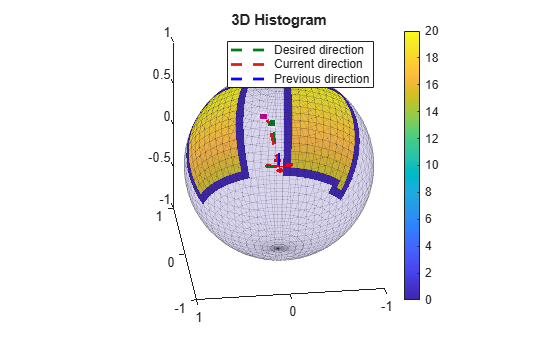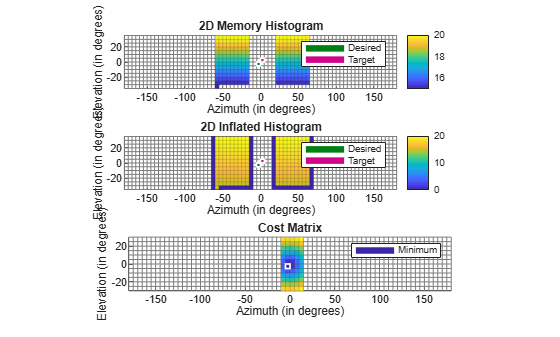# controllerVFH3D

Avoid obstacles using 3D vector field histogram

Since R2022b

## Description

The `controllerVFH3D` System object™ enables a UAV to avoid obstacles, based on sensor data, by using 3D vector field histograms (3DVFH). The object computes an obstacle-free direction using the sensor-data-based positions of obstacles, the UAV position, the UAV orientation, and a target position.

`controllerVFH3D` uses the 3DVFH+ algorithm to compute an obstacle-free direction.

To find an obstacle-free direction:

1. Create the `controllerVFH3D` object and set its properties.

2. Call the object with arguments, as if it were a function.

## Creation

### Syntax

``vfh3D = controllerVFH3D``
``vfh3D = controllerVFH3D(Name=Value)``

### Description

example

````vfh3D = controllerVFH3D` returns a 3-D vector field histogram object, `vfh3D`, that computes a desired direction, a desired yaw, and a status output using the 3DVFH+ algorithm.```
````vfh3D = controllerVFH3D(Name=Value)` returns a vector field histogram object with properties specified by one or more name-value arguments. Properties not specified retain their default values.```

## Properties

expand all

Unless otherwise indicated, properties are nontunable, which means you cannot change their values after calling the object. Objects lock when you call them, and the `release` function unlocks them.

If a property is tunable, you can change its value at any time.

Histogram grid resolution, specified as `1`, `3`, `5`, `6`, `10`, `15`, `18`, `30`, `45`, or `60`. All values are in degrees.

Example: `HistogramResolution=10`

Data Types: `single` | `double`

Histogram window size, specified as a positive odd integer. The histogram window size determines the angular width of an obstacle-free opening in the azimuth and elevation directions. This value is unitless.

Example: `WindowSize=3`

Data Types: `single` | `double`

Threshold for computing the histogram, specified as a positive integer. This value specifies the minimum number of obstacle points that must be in a histogram cell for the cell to be considered as an obstacle. If a cell contains fewer than this number of obstacle points, the cell is considered as obstacle-free.

Example: `HistogramThreshold=2`

Data Types: `single` | `double`

Maximum age of remembered obstacle points, specified as a numeric scalar. This value specifies the number of time steps prior to the current step from which the object remembers the obstacle points.

Example: `MaxAge=1`

Data Types: `single` | `double`

Limits of the range sensor, specified as a vector of the form ```[min max]```, with values in meters.

Example: `DistanceLimits=[0.3 15]`

Data Types: `single` | `double`

Horizontal field of view limits of the range sensor, specified as a vector of the form ```[min max]```, with values in degrees.

Example: `HorizontalSensorFOV=[-50 50]`

Data Types: `single` | `double`

Vertical field of view limits of the range sensor, specified as a vector of the form ```[min max]```, with values in degrees.

Example: `VerticalSensorFOV=[-20 20]`

Data Types: `single` | `double`

Sensor mounting location on the UAV, specified as a vector of the form ```[x y z]```, with values in meters.

Example: `SensorLocation=[0 0 0]`

Data Types: `single` | `double`

Orientation of the sensor mounted on the UAV, specified as a vector of the form ```[roll pitch yaw]```, with values in degrees.

Example: `SensorOrientation=[0 0 0]`

Data Types: `single` | `double`

Radius of the UAV, specified as a numeric scalar in meters. This value specifies the radius of the smallest circle that can circumscribe the UAV. The object uses the vehicle radius to account for vehicle size when computing the obstacle-free direction.

Example: `VehicleRadius=0.5`

Data Types: `single` | `double`

Safety distance between UAV and obstacle, specified as a numeric scalar in meters. This value specifies the space to account for between the UAV and obstacles in addition to the vehicle radius. The object uses both the vehicle radius and safety distance to compute the obstacle-free direction.

Example: `SafetyDistance=2`

Data Types: `single` | `double`

Cost function weight for moving in the target direction, specified as a numeric scalar. To prioritize following a target direction, set this weight to a value greater than the sum of `CurrentDirectionWeight` and `PreviousDirectionWeight`. To remove the target direction from consideration in the cost function, set this weight to `0`.

Example: `TargetDirectionWeight=0`

Data Types: `single` | `double`

Cost function weight for moving in the current direction, specified as a numeric scalar. Higher values of this weight can produce more efficient paths. To remove the current direction from consideration in the cost function, set this weight to `0`.

Example: `CurrentDirectionWeight=0`

Data Types: `single` | `double`

Cost function weight for moving in the previous direction, specified as a numeric scalar. Higher values of this weight can produce smoother paths. To remove the previous direction from consideration in the cost function, set this weight to `0`.

Example: `PreviousDirectionWeight=1`

Data Types: `single` | `double`

## Usage

### Syntax

``[desiredDirection,desiredYaw,status] = vhf3D(position,orientation,obstaclePoints,targetPosition)``

### Description

example

````[desiredDirection,desiredYaw,status] = vhf3D(position,orientation,obstaclePoints,targetPosition)` finds an obstacle-free direction and yaw, using the 3DVFH+ algorithm, for the input UAV position, UAV orientation, sensor-data-based positions of obstacles, and target position. This syntax also returns the status `status` of the obstacle-free direction.```

### Input Arguments

expand all

Position of the UAV, specified as a vector of the form ```[x; y; z]```, in meters.

Example: `[1; 1; 1]`

Data Types: `single` | `double`

Orientation of the UAV, specified as a quaternion vector of the form ```[w; x; y; z]```.

Example: `[1; 0; 0; 0]`

Data Types: `single` | `double`

Positions of the obstacles, specified as an N-by-3 matrix with rows of the form ```[x y z]```, in meters. N is the number of obstacle points.

Example: `[1 1 1; 2 2 2]`

Data Types: `single` | `double`

Target position, specified as a vector of the form ```[x; y; z]```, in meters.

Example: `[2; 3; 4]`

Data Types: `single` | `double`

### Output Arguments

expand all

Desired direction, returned as a vector of the form ```[x; y; z]```, in meters.

Data Types: `single` | `double`

Desired yaw, returned as numeric scalar in the range `[-pi, pi]`, in radians.

Data Types: `single` | `double`

Status of the obstacle-free direction, returned as `0`, `1`, `2`, or `3`.

• `0` — The object finds an obstacle-free direction.

• `1` — The object does not find an obstacle-free direction.

• `2` — The object finds an obstacle-free direction, but the direction is close to an obstacle.

• `3` — The object does not find an obstacle-free direction, and the direction is close to an obstacle.

Data Types: `uint8`

## Object Functions

To use an object function, specify the System object as the first input argument. For example, to release system resources of a System object named `obj`, use this syntax:

`release(obj)`

expand all

 `show` Display 3D vector field histogram
 `step` Run System object algorithm `release` Release resources and allow changes to System object property values and input characteristics `reset` Reset internal states of System object

## Examples

collapse all

Create a `controllerVFH3D` object.

`vfh3D = controllerVFH3D;`

Create obstacles.

```az = [-60:-20 20:60]*(pi/180); el = (-30:30)*(pi/180); [El,Az] = meshgrid(el,az);```

Specify the distances of the obstacles from the sensor, and convert to Cartesian coordinates.

```obstacleDist = linspace(15,20,numel(El(:))); [xSensor,ySensor,zSensor] = sph2cart(Az(:),El(:),obstacleDist');```

Align the sensor and histogram frames.

`vfh3D.SensorOrientation = [-180 0 0];`

Specify the sensor range limits.

`vfh3D.DistanceLimits = [0.2 25];`

Specify the current UAV position and orientation, the locations of obstacles, and the target position for the UAV.

```uavPosition = [0; 0; 0]; uavOrientation = [1; 0; 0; 0]; sensorPoints = [xSensor ySensor zSensor]; targetPosition = [20; 0; 0];```

Compute an obstacle-free direction and desired yaw for the UAV, and return the status of the obstacle-free direction.

```[desiredDirection,desiredYaw,status] = vfh3D(uavPosition, ... uavOrientation, ... sensorPoints, ... targetPosition);```

Visualize the default histogram of the calculated direction.

```show(vfh3D) axis equal```Visualize the 2D memory histogram, 2D inflated histogram, and cost matrix.

```figure ax(1) = subplot(3,1,1); ax(2) = subplot(3,1,2); ax(3) = subplot(3,1,3); show(vfh3D, ... Parent=ax, ... PlotsToShow=["2D Memory Histogram","2D Inflated Histogram","Cost Matrix"]) axis(ax,"equal") xlim(ax,"tight")```## Version History

Introduced in R2022b